#### Chapter 15 Areas of Parallelogram and Triangles R.D. Sharma Solutions for Class 9th Exercise 15.3

Exercise 15.3

1. In Fig. 15.74, compute the area of quadrilateral ABCD.Solution2. In Fig. 15.75, PQRS is a square and T and U are, respectively, the mid-points of PS and QR. Find the area of Δ OTS if PQ = 8 cm.

Solution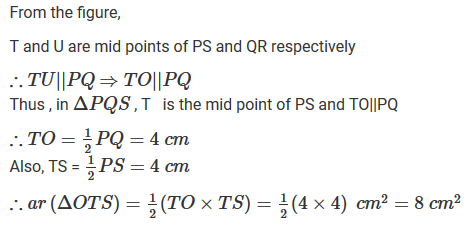3. Compute the area of trapezium PQRS in figure

Solution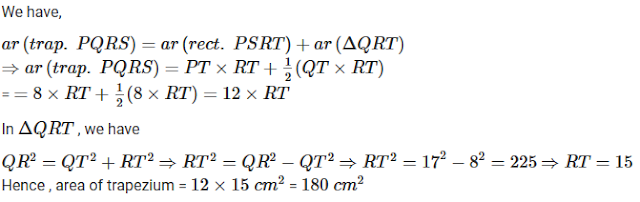4. In Fig. 15.77, ∠AOB = 90°, AC = BC, OA = 12 cm and OC = 6.5 cm. Find the area of Δ AOB.

Solution5. In Fig. 15.78, ABCD is a trapezium in which AB = 7 cm, AD = BC = 5 cm, DC = x cm, and distance between AB and DC is 4 cm. Find the value of x and area of trapezium ABCD.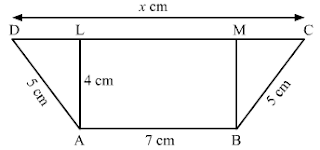Solution6. In Fig. 15.79, OCDE is a rectangle inscribed in a quadrant of a circle of radius 10 cm. If OE = 2√5, find the area of the rectangle.

Solution7. In Fig. 15.80, ABCD is a trapezium in which AB || DC. Prove that:
ar(Δ AOD) = ar(Δ BOC)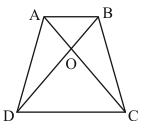Solution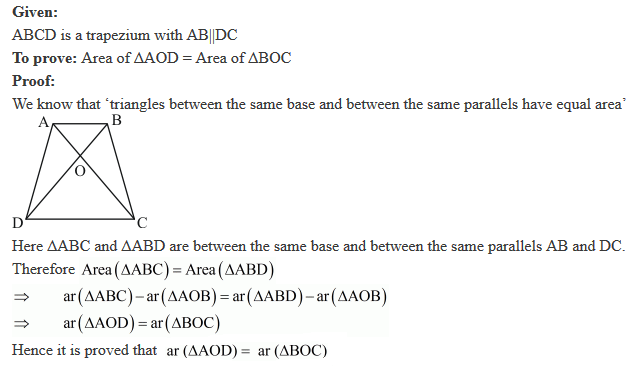8. In Fig. 15.81, ABCD, ABFE and CDEF are parallelograms. Prove that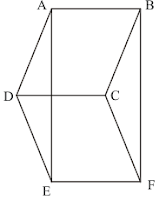Solution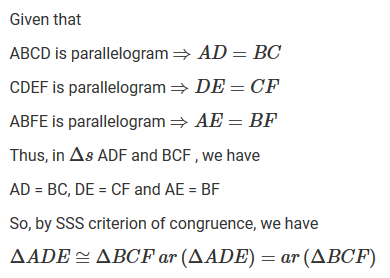9. Diagonals AC and BD of a quadrilateral ABCD intersect each other at P. Show that:
ar(Δ APB) ✕ ar (Δ CPD) = ar (Δ APD) ✕ ar (Δ BPC)

Solution10. In Fig. 15.82, ABC and ABD are two triangles on the base AB. If line segment CD is bisected by AB at O, show that ar (Δ ABC) = ar (Δ ABD)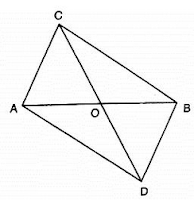Solution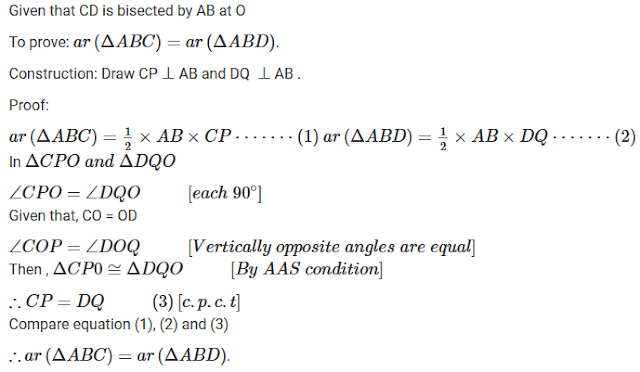11. If P is any point in the interior of a parallelogram ABCD, then prove that area of the triangle APB is less than half the area of parallelogram.

Solution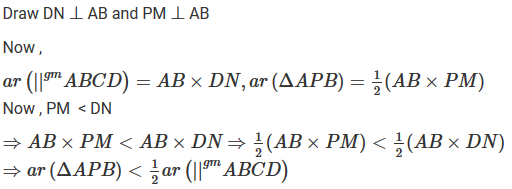12. If AD is a median of a triangle ABC, then prove that triangles ADB and ADC are equal in area. If G is the mid-point of median AD, prove that ar(Δ BGC)= 2 ar (Δ AGC).

Solution13. A point D is taken on the side BC of a ΔABC such that BD = 2DC. Prove that ar (Δ ABD) = 2 ar (Δ ADC).

Solution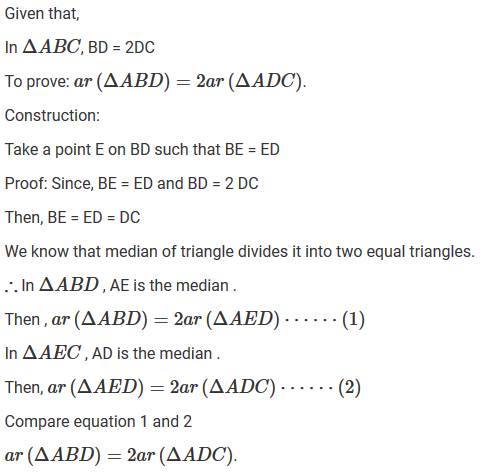14. ABCD is a parallelogram whose diagonals intersect at O. If P is any point on BO, prove that:
(i) ar (Δ ADO) = ar(Δ CDO)
(ii) ar (Δ ABP) = ar (Δ CBP).

Solution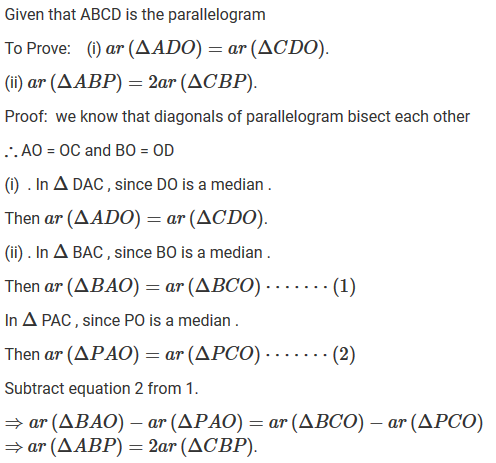15. ABCD is a parallelogram in which BC is produced to E such that CE= BC. AE intersects CD at F.
(i) Prove that ar(△ADF) = ar(△ECF)
(ii) If the area of △DFB = 3cm2, find the area of ∥gm ABCD.

Solution16. ABCD is a parallelogram whose diagonals AC and BD intersect at O . A line through O intersects AB at P and DC at Q. Prove that ar(ΔPOA)=ar(ΔQOC).

Solution17. ABCD is a parallelogram. E is a point on BA such that BE = 2EA and F is point on DC such that DF = 2FC. Prove that AECF is a parallelogram whose area is one third of the area of parallelogram ABCD.

Solution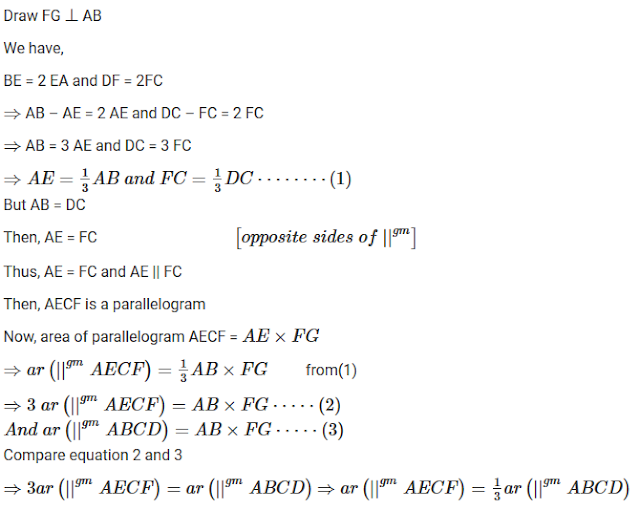18. In a △ABC, P and Q are respectively the mid-points of AB and BC and R is the mid-point of AP. Prove that:
(i) ar(△PBQ) = ar(△ARC)
(ii) ar(△PRQ) = 1/2 ar(△ARC)
(iii) ar(△RQC) = 3/8 ar(△ABC)

Solution19. ABCD is a paralleogram, G is the point on AB such that AG = 2 GB, E is a point of DC such that CE = 2 DE and F is the point of BC such that BF = 2 FC. Prove that:
(ii) ar (△EGB) = 1/6 ar(ABCD)
(iii) ar(△EFC) = 1/2 ar(△EBF)
(iv) ar(△EBG) = ar(△EFC)
(v) Find what portion of the area of the parallelogram is the area of △EFG.

Solution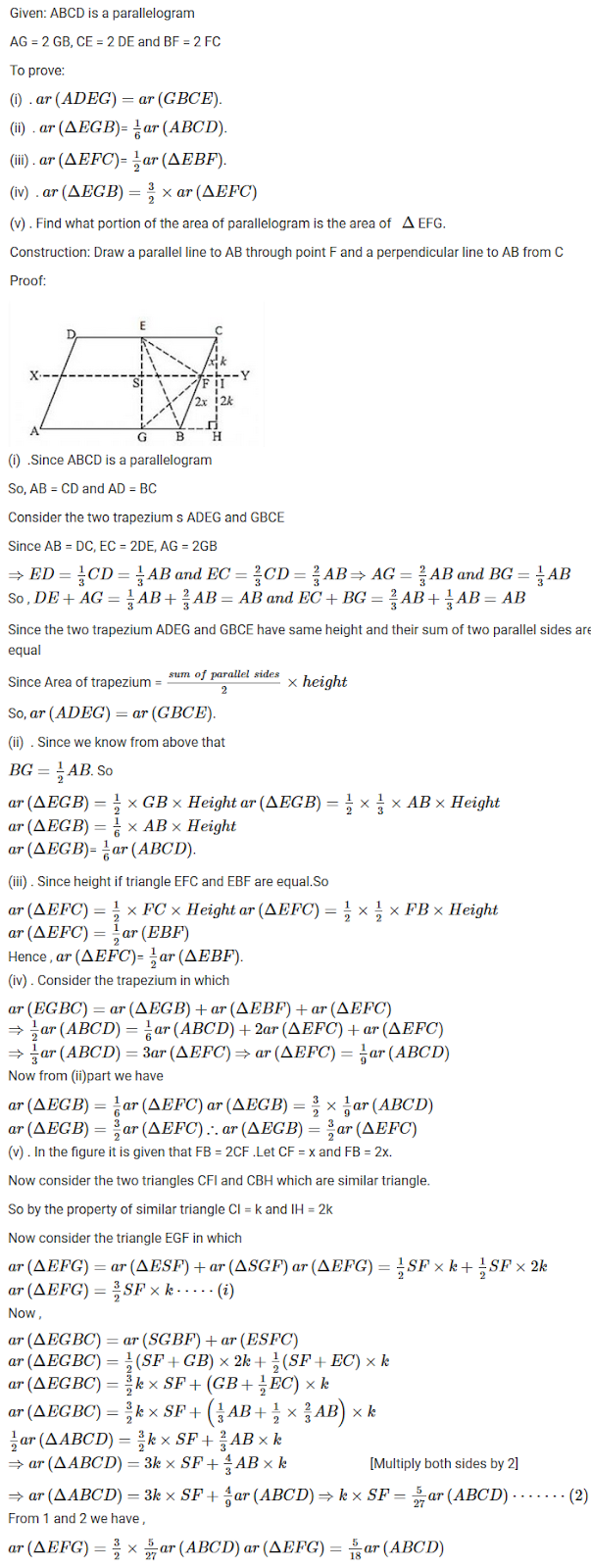20. In Fig. 15.83, CD || AE and CY || BA.
(i) Name a triangle equal in area of ΔCBX
(ii) Prove that ar (Δ ZDE) = ar (Δ CZA)
(iii) Prove that ar (BCZY) = ar (Δ EDZ).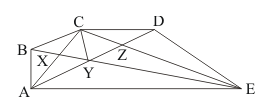Solution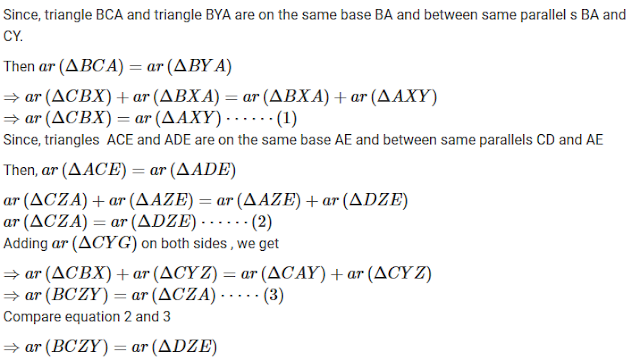21. In Fig. 15.84, PSDA is a parallelogram in which PQ = QR = RS and AP || BQ || CR. Prove that ar (PQE) = ar (Δ CFD).

Solution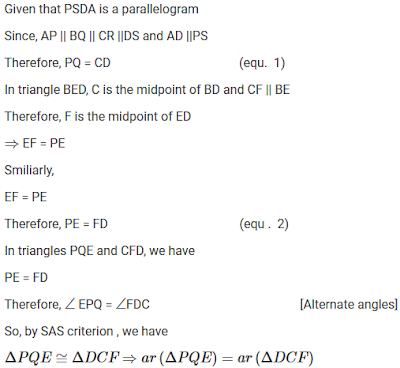22. In Fig. 15.85, ABCD is a trapezium in which AB∥DC and DC = 40 cm and AB = 60 cm. If X and Y are respectively the mid-points of AD and BC, prove that:
(i) XY = 50 cm
(ii) DCYX is a trapezium.
(iii) ar (trap. DCXY) = 9/11 ar (trap. XYBA)

Solution23. In Fig. 15.86, ABC and BDE are two equilateral triangles such that D is the mid-point of BC. AE intesects BC in F. Prove that:
(i) ar(△BDE) = 1/4 ar(△ABC)
(ii) ar(△BDE) = 1/2 ar(△BAE)
(iii) ar(△BFE) = ar(△AFD)
(iv) ar(△ABC) = 2 ar(△BEC)
(v) ar(△FED) = 1/8 ar(△AFC)
(vi) ar(△BFE) = 2 ar(△EFD)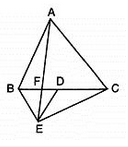Solution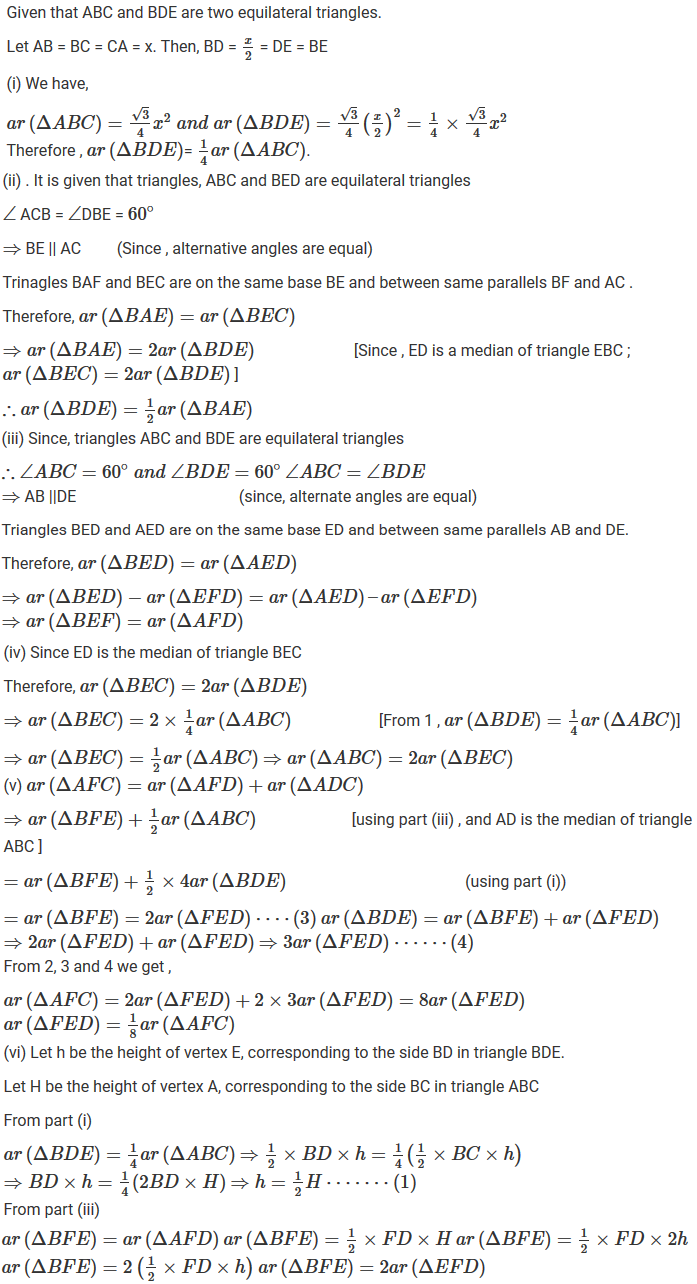24. D is the mid-point of side BC of △ABC and E is the mid-point of BD. If O is the mid-point of AE, prove that ar(△BOE) = 1/8 ar(△ABC)

Solution25. In Fig. 15.87, X and Y are the mid-points of AC and AB respectively, QP || BC and CYQ and BXP are straight lines. Prove that ar (Δ ABP) = ar (Δ ACQ).Solution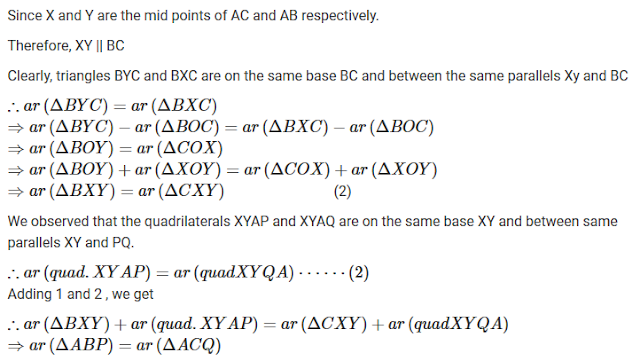26. In Fig. 15.88, ABCD and AEFD are two parallelograms. Prove that

(i) PE = FQ
(ii) ar (Δ APE): ar (ΔPFA) = ar Δ (QFD) : ar (Δ PFD)
(iii) ar (Δ PEA) = ar (Δ QFD).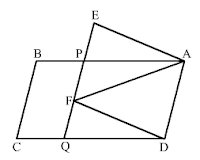Solution28. In a △ABC, if L and M are points on AB and AC respectively such that LM∥BC. Prove that:
(i) ar(△LCM) = ar(△LBM)
(ii) ar(△LBC) = ar(△MBC)
(iii) ar(△ABM) = ar(△ACL)
(ii) ar(△LOB) = ar(△MOC)

Solution29. In figure, D and E are two points on BC such that BD = DE = EC. Show that:Solution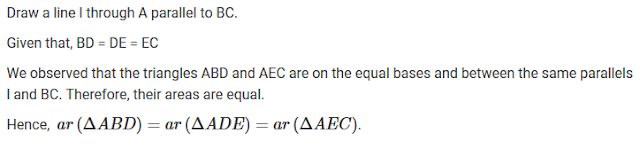30 . If Fig. 15.91, ABC is a right triangle right angled at A, BCED, ACFGand ABMN are squares on the sides BC, CA and AB respectively. Line segment AX ⊥ DE meets BC at Y.
Show that
(i) ΔMBC ≅ Δ ABD
(ii) ar (BYXD) = 2 ar (Δ MBC)
(iii) ar (BYXD) = ar (ABMN)
(iv) Δ FCB ≅ Δ ACE
(v) ar (CYXE) = 2 ar (ΔFCB)
(vi) ar (CYXE) = ar (ACFG)
(vii) ar (BCED) = ar (ABMN) + ar (ACFG)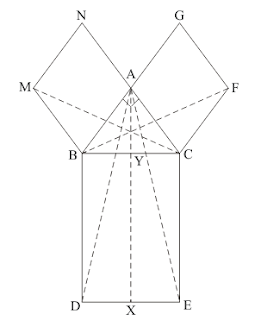Solution